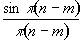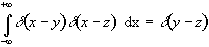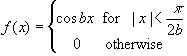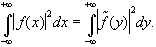# 1.[2pt]Series expansion

Ohanian writes on page 28 that the “factorequals zero if n ≠ m, and it equals 1 if n=m.  State the assumptions under which this is true and then prove Ohanian’s statement.

# 2.[1pt+2pt+2pt+3pt]Dirac Delta “function”

Prove the following identities:

(i)                  d(-x) = d(x)

(ii)                x d(x-a) = a d(x-a)

(iii)               d(cx) = d(x)/|c| for c ≠ 0

(iv)# 3.[5pt]Fourier series

Consider the functionIn the interval -p/(2b) < x < p/(2b)) represent the function f as a fourier series and evaluate the coefficients.  Plot the fourier series of order n=1, n=2, n=3 (i.e. truncate the summation after the first, second and third terms, respectively).

# 4.[5pt]Fourier transform

Find the fourier transformof the same function f(x) considered in problem 3 and plot the fourier transform.  Show explicitly that